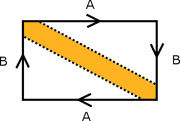## FANDOM

36 PagesPublish or Perish, 1970 (first ed.), 1979 (second ed.), 1999 (third ed.)

"There are two main premises on which these notes are based. the first premise is that it is absurdly inefficient to eschew the modern language of manifolds, bundles, forms, etc., which was developed precisely in order to rigorize the concepts of classical differential geometry. Rephrasing everything in more elementary terms involves incredible contortions which are not only unnecessary, but misleading. The work of Gauss, for example, which uses infinitesimals throughout, is most naturally rephrased in terms of differentials, even if it is possible to rewrite it in terms of derivatives... The second premise for these notes is that in order for an introduction to differential geometry to expose the geometric aspect of the subject, an historical approach is necessary; there is no point in introducing the curvature tensor without explaining how it was invented and what it has to do with curvature."

# Chapter 1. ManifoldsEdit

## Elementary Properties of ManifoldsEdit

• Proof of Theorem 2: The set of all such r > 0 is an interval. In particular, it's an interval of the form (0, s) or (0, s]. This follows because of the elementary result of general topology that a closed subset of a compact space is closed.
• Proof of Theorem 2: Now let $x_0 \in X$ and consider the compact sets $A_1 = \{x_0\}$, $A_{n+1} = A_n'$. Their union A is clearly open. How is this clear?
•Definition of $\mathbb{P}^2$: The dotted lines in this picture are the key to understanding $\mathbb{P}^2$. At least in the second edition, what appears to be a single, serpentine dotted line is actually two dotted lines and some kind of coloring indicated in between, like in the diagram floating to the right. The orange piece is a Möbius strip, the white part is a disc, and together they make a projective plane.
• The metric on $\mathbb{P}^2$: To see where this came from, consider the Veronese embedding $v : \mathbb{P}^2 \to \mathbb{P}^5$ given by $v([x:y:z]) = [ x^2 : x y : y^2 : x z : y z : z^3 ]$. We can check that composing with a projection to get $[ x y : x z : y z : x^2 + y^2 + z^2 : x^2 + 2 y^2 + 3 z^2 ]$ doesn't lose injectivity. Further, since we're working over the reals, $x^2 + y^2 + z^2$ is nonzero everywhere, so we can divide through and get a map $\mathbb{P}^2 \to \mathbb{A}^4$ taking [x:y:z] to $\left( \frac{x y}{x^2 + y^2 + z^2},\; \frac{x z}{x^2 + y^2 + z^2},\; \frac{y z}{x^2 + y^2 + z^2},\; \frac{x^2 + 2 y^2 + 3 z^3}{x^2 + y^2 + z^2}\right)$. Since each numerator and denominator is homogeneous of second degree, this map can be pulled back to a map $S^2 \to \mathbb{A}^4$. And note that, on $S^2$, we have $x^2 + y^2 + z^2 = 1$.

# Chapter 2. Differentiable StructuresEdit

## Partial derivativesEdit

• Definition of $\theta(x,y)$. At least in the second edition, one of the not-equals should be an equal sign.

## Critical pointsEdit

• Definition of critical point. p is a critical point of f if the rank of f at p is less than n (the dimension of the image N). "Image" here is probably the wrong word, as illustrated in the example on the next page. "Codomain" would be more appropriate.
• Definition of measure zero. A rectangle here refers to an n-dimensional box.

# Chapter 3. The Tangent BundleEdit

## The tangent space of $\mathbb{R}^n$Edit

• After the definition of tangent vector: Notice that the tangent vector of c at t is the same as $c_\ast(1_t)$... The at-first confusing notation $1_t$ here is an example of the notation for tangent vector at a point, and refers to $(t, 1) \in {\rm T}\mathbb{R}^m$, "1" being an acceptable name for a vector as the tangent space at t is one-dimensional.